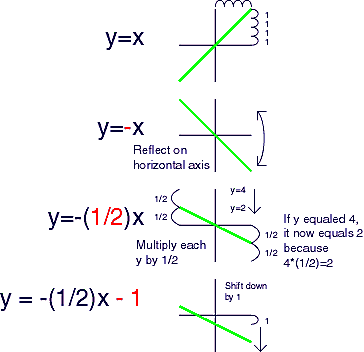### Squishy Function Graphs

by T. Ford - Email me if you find any errors.

Simply:
 Step 1:Pick Function Graph Step 2:Choose an Action GenericFormula Adjustment```Reflect on X axis Reflect on Y axis Shift left Shift right Shift up Shift down Shrink vertically Stretch vertically Shrink horizontally Stretch horizontally``` ```y=-f(x) y=f(-x) y=f(x+b) y=f(x-b) y=f(x)+b y=f(x)-b y=af(x), 01 y=f(cx), c>1 y=f(cx), 0```Using the above, variations on functions can be split apart.

Example 1:
y = -a|-cx + b| + d
Graph: y = |x|
Reflect on x axis. (first - sign) (make it upside down!)
If a>1, stretch vertically.
If a<1, shrink vertically.
Reflect on the y axis. (second - sign) (note: no change for absolute value graph)
If c>1, shrink horizontally.
If c<1, stretch horizontally.
Shift it left b. (for the + b inside the absolute value function)
Shift it up d. (for the + d outside the absolute value function)

Example 2:
y = -a(1/(cx - b)) + d
Graph: y = 1/x
Reflect on x axis. (first - sign) (make it upside down!)
If a>1, stretch vertically.
If a<1, shrink vertically.
Do not reflect on the y axis. (missing - sign on c)
If c>1, shrink horizontally.
If c<1, stretch horizontally.
Shift it right b. (for the - b inside the absolute value function)
Shift it up d. (for the + d outside the absolute value function)

Example 3:
y = -(1/2)x - 1
Graph y=x
Reflect on x axis. (first - sign) (make it upside down!)
Shrink vertically.  ((1/2)<1)
No modifications to x, so no y axis reflection, horizontal stretching,
or horizontal shifting.
A modification to x might look like: y = -(1/2)(2x+3) - 1
Shift it down 1. (for the - 1 outside the linear function)
```### Some squishy notes: